# How to Convert Integers to Floats in Pandas DataFrame?

• Last Updated : 25 Aug, 2020

Pandas Dataframe provides the freedom to change the data type of column values. We can change them from Integers to Float type, Integer to String, String to Integer, etc.

There are 2 methods to convert Integers to Floats:

Method 1: Using DataFrame.astype() method

Syntax :

```DataFrame.astype(dtype, copy=True, errors=’raise’, **kwargs)
```

Example 1: Converting one column from int to float using DataFrame.astype()

## Python3

 `# importing pandas library``import` `pandas as pd`` ` `# Initializing the nested list with Data set``player_list ``=` `[[``'M.S.Dhoni'``, ``36``, ``75``, ``5428000``, ``176``], ``               ``[``'A.B.D Villers'``, ``38``, ``74``, ``3428000``, ``175``], ``               ``[``'V.Kholi'``, ``31``, ``70``, ``8428000``, ``172``],``               ``[``'S.Smith'``, ``34``, ``80``, ``4428000``, ``180``], ``               ``[``'C.Gayle'``, ``40``, ``100``, ``4528000``, ``200``],``               ``[``'J.Root'``, ``33``, ``72``, ``7028000``, ``170``], ``               ``[``'K.Peterson'``, ``42``, ``85``, ``2528000``, ``190``]]`` ` `# creating a pandas dataframe``df ``=` `pd.DataFrame(player_list, columns``=``[``                  ``'Name'``, ``'Age'``, ``'Weight'``, ``'Salary'``, ``'Strike_rate'``])`` ` `# lets find out the data type ``# of 'Weight' column``print``(df.dtypes)`

Output: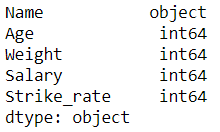Let’s convert weight type to float

## Python3

 `# Now we will convert it from 'int' to 'float' type ``# using DataFrame.astype() function``df[``'Weight'``] ``=` `df[``'Weight'``].astype(``float``)`` ` `print``()`` ` `# lets find out the data type after changing``print``(df.dtypes)`` ` `# print dataframe. ``df`

Output: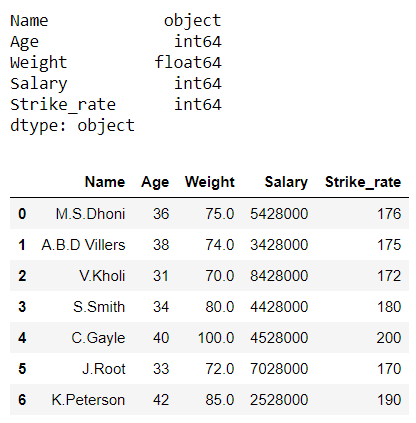In the above example, we change the data type of column ‘Weight‘ from ‘int64’ to ‘float64’.

Example 2: Converting more than one column from int to float using DataFrame.astype()

## Python3

 `# importing pandas library``import` `pandas as pd`` ` `# Initializing the nested list with Data set``player_list ``=` `[[``'M.S.Dhoni'``, ``36``, ``75``, ``5428000``, ``176``], ``               ``[``'A.B.D Villers'``, ``38``, ``74``, ``3428000``, ``175``],``               ``[``'V.Kholi'``, ``31``, ``70``, ``8428000``, ``172``],``               ``[``'S.Smith'``, ``34``, ``80``, ``4428000``, ``180``],``               ``[``'C.Gayle'``, ``40``, ``100``, ``4528000``, ``200``],``               ``[``'J.Root'``, ``33``, ``72``, ``7028000``, ``170``], ``               ``[``'K.Peterson'``, ``42``, ``85``, ``2528000``, ``190``]]`` ` `# creating a pandas dataframe``df ``=` `pd.DataFrame(player_list, columns``=``[``                  ``'Name'``, ``'Age'``, ``'Weight'``, ``'Salary'``, ``'Strike_rate'``])`` ` `# lets find out the data type of 'Age' ``# and 'Strike_rate' columns``print``(df.dtypes)`

Output: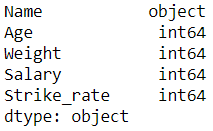Let’s convert age and strike_rate to float type

## Python3

 `# now Pass a dictionary to astype() function  ``# which contains two columns ``# and hence convert them from int to float type``df ``=` `df.astype({``"Age"``:``'float'``, ``"Strike_rate"``:``'float'``}) ``print``()`` ` `# lets find out the data type after changing``print``(df.dtypes)`` ` `# print dataframe. ``df `

Output: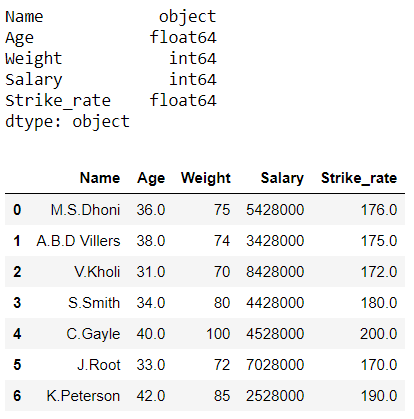In the above example, we change the data type of columns ‘Age‘ and ‘Strike_rate’ from ‘int64’ to ‘float64’.

Method 2: Using pandas.to_numeric() method

Syntax

```pandas.to_numeric(arg, errors=’raise’, downcast=None)
```

Example 1:  Converting a single column from int to float using pandas.to_numeric()

## Python3

 `# importing pandas library``import` `pandas as pd`` ` `# Initializing the nested list with Data set``player_list ``=` `[[``'M.S.Dhoni'``, ``36``, ``75``, ``5428000``, ``176``], ``4``               ``[``'A.B.D Villers'``, ``38``, ``74``, ``3428000``, ``175``], ``               ``[``'V.Kholi'``, ``31``, ``70``, ``8428000``, ``172``],``               ``[``'S.Smith'``, ``34``, ``80``, ``4428000``, ``180``], ``               ``[``'C.Gayle'``, ``40``, ``100``, ``4528000``, ``200``],``               ``[``'J.Root'``, ``33``, ``72``, ``7028000``, ``170``], ``               ``[``'K.Peterson'``, ``42``, ``85``, ``2528000``, ``190``]]`` ` `# creating a pandas dataframe``df ``=` `pd.DataFrame(player_list, columns``=``[``                  ``'Name'``, ``'Age'``, ``'Weight'``, ``'Salary'``, ``'Height'``])`` ` `# lets find out the data type of ``# 'Weight' column``print``(df.dtypes)`

Output: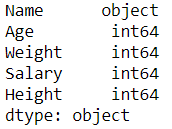Let’s convert weight from int to float

## Python3

 `# Now we will convert it from 'int' to 'float' type``# using pandas.to_numeric()``df[``'Weight'``] ``=` `pd.to_numeric(df[``'Weight'``], downcast``=``'float'``)``print``()`` ` `# lets find out the data type after changing``print``(df.dtypes)`` ` `# print dataframe. ``df `

Output: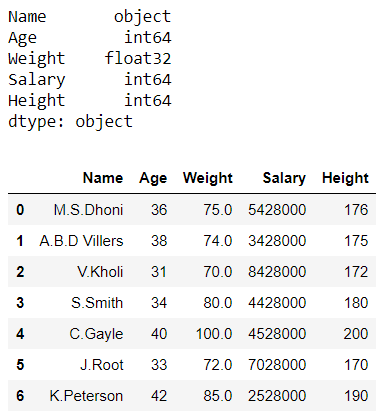In the above example, we change the data type of column ‘Weight‘ from ‘int64’ to ‘float32’.

Example 2:

## Python3

 `# importing pandas library``import` `pandas as pd`` ` `# Initializing the nested list with Data set``player_list ``=` `[[``'M.S.Dhoni'``, ``36``, ``75``, ``5428000``, ``176``], ``               ``[``'A.B.D Villers'``, ``38``, ``74``, ``3428000``, ``175``], ``               ``[``'V.Kholi'``, ``31``, ``70``, ``8428000``, ``172``],``               ``[``'S.Smith'``, ``34``, ``80``, ``4428000``, ``180``], ``               ``[``'C.Gayle'``, ``40``, ``100``, ``4528000``, ``200``],``               ``[``'J.Root'``, ``33``, ``72``, ``7028000``, ``170``], ``               ``[``'K.Peterson'``, ``42``, ``85``, ``2528000``, ``190``]]`` ` `# creating a pandas dataframe``df ``=` `pd.DataFrame(player_list, columns``=``[``                  ``'Name'``, ``'Experience'``, ``'Weight'``, ``'Salary'``, ``'Height'``])`` ` `# lets find out the data type of ``# 'Experience' and 'Height' columns``print``(df.dtypes)`

Output: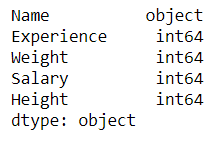Let’s convert experience and height from int to float

## Python3

 `# Now we will convert them from 'int' to 'float' type``# using pandas.to_numeric()``df[``'Experience'``] ``=` `pd.to_numeric(df[``'Experience'``], downcast``=``'float'``)``df[``'Height'``] ``=` `pd.to_numeric(df[``'Height'``], downcast``=``'float'``)`` ` `print``()`` ` `# lets find out the data type after changing``print``(df.dtypes)`` ` `# print dataframe. ``df`

Output: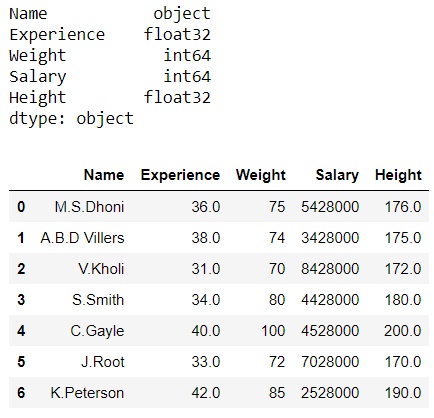In the above example, we change the data type of columns ‘Experience’ and ‘Height’  from ‘int64’ to ‘float32’.

My Personal Notes arrow_drop_up